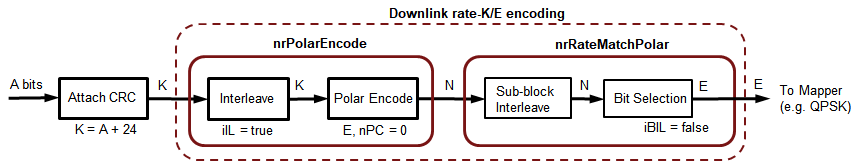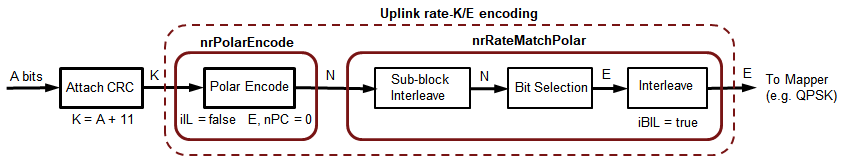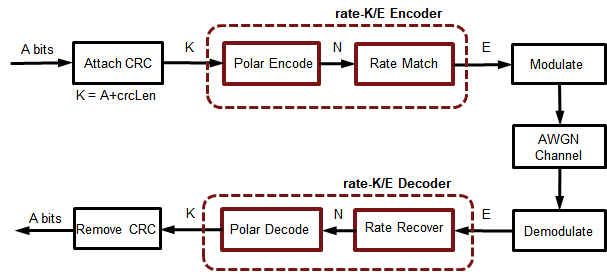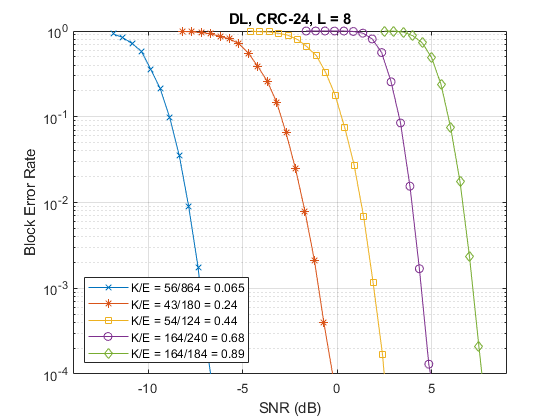# 5G New Radio Polar Coding

This example highlights the new polar channel coding technique chosen for 5G New Radio (NR) communications system. Of the two main types of code constructions specified by 3GPP, this example models the CRC-Aided Polar (CA-Polar) coding scheme. This example describes the main components of the polar coding scheme with individual components for code construction, encoding and decoding along-with rate-matching. It models a polar-coded QPSK-modulated link over AWGN and presents Block-Error-Rate results for different message lengths and code rates for the coding scheme.

### Introduction

The selection of polar codes as the channel coding technique for control channels for 5G NR communications system has proven the merits of Arikan's [ 1 ] discovery and will establish their application in commercial systems [ 6 ]. Based on the concept of channel polarization, this new coding family is capacity achieving as opposed to just capacity approaching. With better or comparable performance than LDPC and turbo codes, it supersedes the tail-biting convolutional codes used in LTE systems for control channels. It is applied for downlink and uplink control information (DCI/UCI) for the enhanced mobile broadband (eMBB) use case, as well as the broadcast channel (BCH). Alternatively, the channel coding scheme for data channels for eMBB is specified to be flexible LDPC for all block sizes.

This example highlights the components to enable a polar coding downlink simulation using QPSK modulation over an AWGN channel. In the following sections, the individual polar coding components are further detailed.

```s = rng(100); % Seed the RNG for repeatability ```

Specify the code parameters used for a simulation.

```% Code parameters K = 54; % Message length in bits, including CRC, K > 30 E = 124; % Rate matched output length, E <= 8192 EbNo = 0.8; % EbNo in dB L = 8; % List length, a power of two, [1 2 4 8] numFrames = 10; % Number of frames to simulate linkDir = 'DL'; % Link direction: downlink ('DL') OR uplink ('UL') ```

### Polar Encoding

The following schematic details the transmit-end processing for the downlink, with relevant components and their parameters highlighted.For the downlink, the input bits are interleaved prior to polar encoding. The CRC bits appended at the end of the information bits are thus distributed for the CA-Polar scheme. This interleaving is not specified for the uplink.

The polar encoding uses an SNR-independent method where the reliability of each subchannel is computed offline and the ordered sequence stored for a maximum code length [ 6 ]. The nested property of polar codes allows this sequence to be used for any code rate and all code lengths smaller than the maximum code length.

This sequence is computed for given rate-matched output length, `E`, and information length, `K`, by the function `nrPolarEncode`, which implements the non-systematic encoding of the input `K` bits.

```if strcmpi(linkDir,'DL') % Downlink scenario (K >= 36, including CRC bits) crcLen = 24; % Number of CRC bits for DL, Section 5.1,  poly = '24C'; % CRC polynomial nPC = 0; % Number of parity check bits, Section 5.3.1.2,  nMax = 9; % Maximum value of n, for 2^n, Section 7.3.3,  iIL = true; % Interleave input, Section 5.3.1.1,  iBIL = false; % Interleave coded bits, Section 5.4.1.3,  else % Uplink scenario (K > 30, including CRC bits) crcLen = 11; poly = '11'; nPC = 0; nMax = 10; iIL = false; iBIL = true; end ```

The following schematic details the transmit-end processing for the uplink, for a payload size greater than `19` bits and no code-block segmentation, with relevant components and their parameters highlighted.### Rate Matching and Rate Recovery

The polar encoded set of bits (`N`) are rate-matched to output the specified number of bits (`E`) for resource element mapping [ 7 ]. The coded bits are sub-block interleaved and passed to a circular buffer of length `N`. Depending on the desired code rate and selected values of `K`, `E`, and `N`, either of repetition (`E >= N`), and puncturing or shortening (`E < N`) is realized by reading the output bits from the buffer.

• For puncturing, `E` bits are taken from the end

• For shortening, `E` bits are taken from the start

• For repetition, `E` bits are repeated modulo `N`.

For the downlink, the selected bits are passed on to the modulation mapper, while for the uplink, they are further interleaved prior to mapping. The rate-matching processing is implemented by the function `nrRateMatchPolar`.

At the receiver end, rate recovery is accomplished for each of the cases

• For puncturing, corresponding LLRs for the bits removed are set to zero

• For shortening, corresponding LLRs for the bits removed are set to a large value

• For repetition, the set of LLRs corresponding to first `N` bits are selected.

The rate-recovery processing is implemented by the function `nrRateRecoverPolar`.

```R = K/E; % Effective code rate bps = 2; % bits per symbol, 1 for BPSK, 2 for QPSK EsNo = EbNo + 10*log10(bps); snrdB = EsNo + 10*log10(R); % in dB noiseVar = 1./(10.^(snrdB/10)); % Channel chan = comm.AWGNChannel('NoiseMethod','Variance','Variance',noiseVar); ```

### Polar Decoding

The implicit CRC encoding of the downlink (DCI or BCH) or uplink (UCI) message bits dictates the use of the CRC-Aided Successive Cancellation List Decoding (CA-SCL) [ 3 ] as the channel decoder algorithm. It is well known that CA-SCL decoding can outperform turbo or LDPC codes [ 4 ] and this was one of the major factors in the adoption of polar codes by 3GPP.

Tal & Vardy [ 2 ] describe the SCL decoding algorithm in terms of likelihoods (probabilities). However, due to underflow, the inherent computations are numerically unstable. To overcome this issue, Stimming et.al. [ 5 ] offer the SCL decoding solely in the log-likelihood ratio (LLR) domain. The list decoding is characterized by the `L` parameter, which represents the number of most likely decoding paths retained. At the end of the decoding, the most likely code-path among the `L` paths is the decoder output. As `L` is increased, the decoder performance also improves, however, with a diminishing-returns effect.

For an input message which is concatenated with a CRC, CA-SCL decoding prunes out any of the paths for which the CRC is invalid, if at least one path has the correct CRC. This additional insight in the final path selection improves the performance further, when compared to SCL decoding. For the downlink, a CRC of 24 bits is used, while for the uplink CRCs of 6 and 11 bits are specified, which vary on the value of `K`.

The decoder is implemented by the function `nrPolarDecode`, which supports all three CRC lengths. The decoder function also accounts for the input bit interleaving specified at the transmitter for the downlink, prior to outputting the decoded bits.

```% Error meter ber = comm.ErrorRate; ```

### Frame Processing Loop

This section shows how the prior described components for polar coding are used in a Block Error Rate (BLER) simulation. The simulation link is highlighted in the following schematic.For each frame processed, the following steps are performed:

• `K-crcLen` random bits are generated,

• A CRC is computed and appended to these bits

• The CRC appended bits are polar encoded to the mother code block length

• Rate-matching is performed to transmit `E` bits

• The `E` bits are QPSK modulated

• White Gaussian Noise of specified power is added

• The noisy signal is soft QPSK demodulated to output LLR values

• Rate recovery is performed accounting for either of puncturing, shortening or repetition

• The recovered LLR values are polar decoded using the CA-SCL algorithm, including deinterleaving.

• Off the decoded `K` bits, the first `K-crcLen` bits are compared with those transmitted to update the BLER and bit-error-rate (BER) metrics.

At the end of the simulation, the two performance indicators, BLER and BER, are reported.

```numferr = 0; for i = 1:numFrames % Generate a random message msg = randi([0 1],K-crcLen,1); % Attach CRC msgcrc = nrCRCEncode(msg,poly); % Polar encode encOut = nrPolarEncode(msgcrc,E,nMax,iIL); N = length(encOut); % Rate match modIn = nrRateMatchPolar(encOut,K,E,iBIL); % Modulate modOut = nrSymbolModulate(modIn,'QPSK'); % Add White Gaussian noise rSig = chan(modOut); % Soft demodulate rxLLR = nrSymbolDemodulate(rSig,'QPSK',noiseVar); % Rate recover decIn = nrRateRecoverPolar(rxLLR,K,N,iBIL); % Polar decode decBits = nrPolarDecode(decIn,K,E,L,nMax,iIL,crcLen); % Compare msg and decoded bits errStats = ber(double(decBits(1:K-crcLen)), msg); numferr = numferr + any(decBits(1:K-crcLen)~=msg); end disp(['Block Error Rate: ' num2str(numferr/numFrames) ... ', Bit Error Rate: ' num2str(errStats(1)) ... ', at SNR = ' num2str(snrdB) ' dB']) rng(s); % Restore RNG ```
```Block Error Rate: 0, Bit Error Rate: 0, at SNR = 0.20002 dB ```

### Results

To get meaningful results, simulations have to be run for a longer duration. Using scripts which encapsulate the above processing into a function that supports C-code generation, the following results for different code rates and message lengths are presented for both link directions with QPSK modulation.The above results were generated by simulating, for each SNR point, up to 1000 frame errors or a maximum of 100e3 frames, whichever occurred first.

The BLER performance results indicate the suitability of polar codes in a communication link and their implicit support for rate-compatibility at the bit-level granularity.

The use of C-code generation tools for the components reduces the execution time, a key concern for simulations. The C-code generation is enabled by MATLAB Coder™.

### Summary and Further Exploration

This example highlights one of the polar coding schemes (CRC-Aided Polar) specified by 3GPP for New Radio control channel information (DCI, UCI) and broadcast channel (BCH). It shows the use of components for all stages of the processing (encoding, rate-matching, rate-recovery and decoding) and uses them in a link with QPSK over an AWGN channel. Highlighted performance results for different code rates and message lengths show agreement to published trends, within parametric and simulation assumption variations.

Explore simple parameter variations (`K`, `E`, `L`) and their effect on BLER performance. The polar coding functions are implemented as open MATLAB® code to enable their application for both downlink/uplink control information and broadcast channel. The CA-Polar scheme is applicable for both

• Downlink, for all message lengths, and

• Uplink, for `K > 30`, with `crcLen = 11`, `nPC = 0`, `nMax = 10`, `iIL = false`, and `iBIL = true`.

Refer to Modeling Downlink Control Information and NR Cell Search and MIB and SIB1 Recovery examples, for the use of polar coding functions within the DCI and BCH functions respectively.

The highlighted polar coding functions also support the Parity-Check polar coding construction and encoding. This is applicable for the uplink with UCI payloads in range `18 <= K <= 25`. This is supported by the uplink control coding functions `nrUCIEncode` and `nrUCIDecode`, which include code-block segmentation as well for appropriate values of `K` and `E`.

### Selected References

1. Arikan, E., "Channel Polarization: A Method for constructing Capacity-Achieving Codes for Symmetric Binary-Input Memoryless Channels," IEEE Transactions on Information Theory, vol. 55, No. 7, pp. 3051-3073, July 2009.

2. Tal, I, and Vardy, A., "List decoding of Polar Codes", IEEE Transactions on Information Theory, vol. 61, No. 5, pp. 2213-2226, May 2015.

3. Niu, K., and Chen, K., "CRC-Aided Decoding of Polar Codes," IEEE Communications Letters, vol. 16, No. 10, pp. 1668-1671, Oct. 2012.

4. Niu, K., Chen, K., and Lin, J.R., "Beyond turbo codes: rate compatible punctured polar codes", IEEE International Conference on Communications, pp. 3423-3427, 2013.

5. Stimming, A. B., Parizi, M. B., and Burg, A., "LLR-Based Successive Cancellation List Decoding of Polar Codes", IEEE Transaction on Signal Processing, vol. 63, No. 19, pp.5165-5179, 2015.

6. 3GPP TS 38.212. "NR; Multiplexing and channel coding (Release 15)." 3rd Generation Partnership Project; Technical Specification Group Radio Access Network.

7. R1-1711729. "WF on circular buffer of Polar Code", 3GPP TSG RAN WG1 meeting NR Ad-Hoc#2, Ericsson, Qualcomm, MediaTek, LGE. June 2017.